Next: Implementation Up: Sturm method Previous: Sturm method   Contents

Mathematical background

Let a polynomial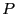and define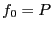,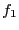be the first derivative of. Then define the sequence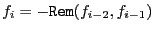where Rem is the remainder of the division of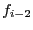by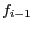. Ifis the degree ofthe last element in the sequence will be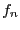. Assume now that we are looking for the number of distinct roots ofin the interval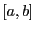with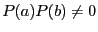. We build two sequences: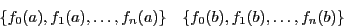Let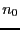be the number of change of sign in the first sequence and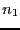be the number of change of sign in the second sequence. Then the number of distinct real roots ofin the intervalis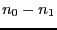if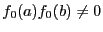. Note that a multiple roots count only for one root with this method.

The drawback of Sturm method is that the absolute value of the coefficients increase quickly when computing the sequence. Numerical rounding errors may then affect the result. The alternate method of Budan-Fourier (see section 5.5.2) is less sensitive to rounding errors although it provides less information than Sturm method.Next: Implementation Up: Sturm method Previous: Sturm method   Contents
Jean-Pierre Merlet 2012-12-20